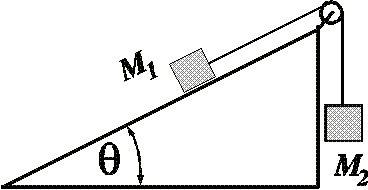# Incline, pulley and friction!

## Homework Statement

The pulley is light and frictionless. Find the mass M1, given that M2 (6.00 kg) is moving downwards and accelerates downwards at 3.61 m/s2, that θ is 30.0°, and that μk is 0.490.

## Homework Equations

F=ma
F||=mg*cos(theta)
Fper=mg*sin(theta)

## The Attempt at a Solution

I have no idea where to start this problem..

I know how to find F|| and Fperendicular when theres no pulley/no friction.. no idea where to start..#### Attachments

Take the freebody diagram of M1... write $$\Sigma\vec{F_x} = m\vec{a_x}$$ and $$\Sigma\vec{F_y} = m\vec{a_y}$$ where x is along the plane, and y is perpendicular to the plane.